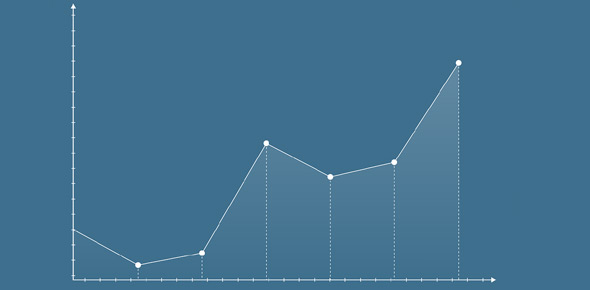# Chart Of Accounts Quiz

15 Questions | Total Attempts: 844SettingsRelated Topics
• 1.
The chart of accounts has a list of:-
• A.

Names of customers and suppliers

• B.

General ledger account names and numbers

• C.

Names of each inventory item

• 2.
Name the five main categories that the ledger accounts are grouped under:-
• A.

Assets, credit cards, liabilities, money, contact cards

• B.

Assets, production, bank register, purchases, sales

• C.

Assets, liabilities, equity, income, expenses

• 3.
Which category usually has (but not always!) the most accounts?
• A.

The banking corporation

• B.

The 10 richest people of the world

• C.

Expenses

• 4.
What equation does the chart of accounts tie-in with?
• A.

Accounting equation

• B.

Wave equation

• C.

The origin of complex numbers

• 5.
You can split a category into manageable sub-groups?
• A.

True

• B.

False

• 6.
Which category do you think an insurance loan would go under?
• A.

Assets

• B.

Liabilities

• C.

Expenses

• 7.
Under which category do you think a new, high cost computer would go ?
• A.

Expenses

• B.

Income

• C.

Assets

• 8.
Which category do you think business Travel & Accommodation costs would go under?
• A.

Expenses

• B.

Liabilities

• C.

Equity

• 9.
Which category do you think wages would go under?
• A.

Equity

• B.

Expenses

• C.

Income

• 10.
Which category do you think the website development costs would go under?
• A.

Assets

• B.

Equity

• C.

Expenses

• 11.
A customer pays a deposit for work not yet started. Where does this go?
• A.

Income

• B.

Assets

• C.

Equity

• 12.
You buy products (stock) to sell to customers – what category do stock purchases go under?
• A.

Expenses

• B.

Liabilities

• C.

Assets

• 13.
The business owner uses business funds to pay his son’s school fees. What category is this?
• A.

Equity

• B.

Liabilities

• C.

Expenses